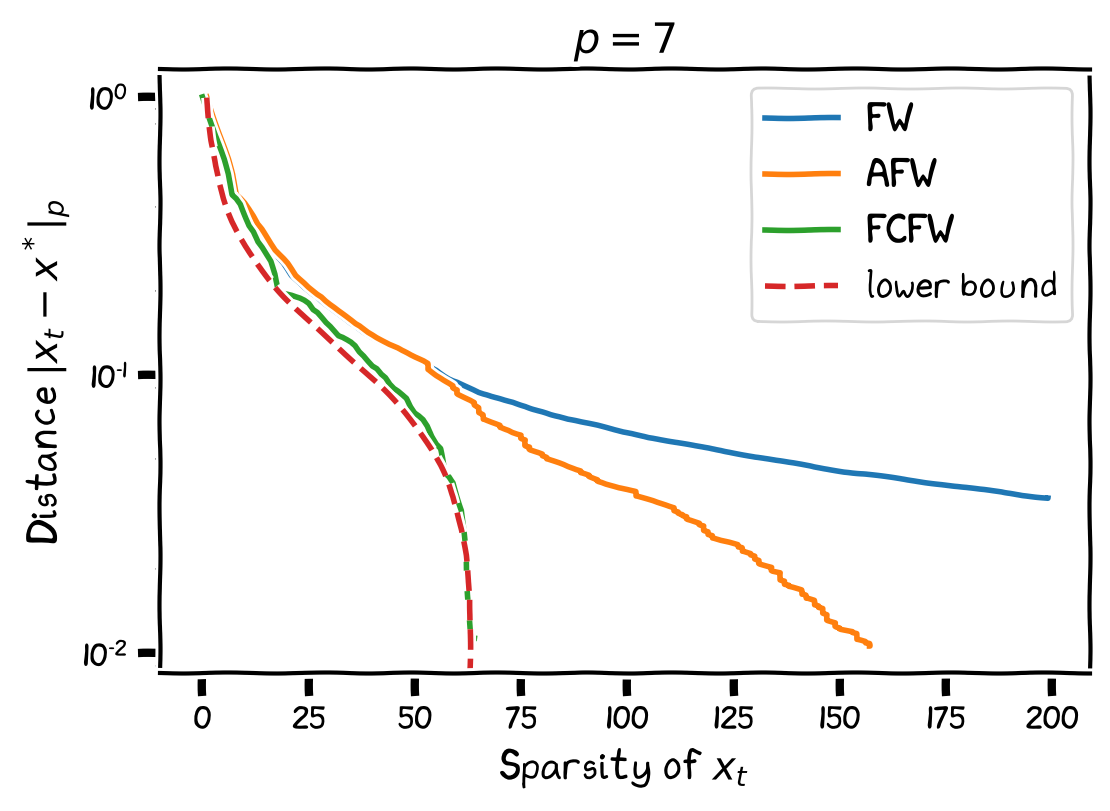TL;DR: This is an informal summary of our recent paper Revisiting the Approximate Carathéodory Problem via the Frank-Wolfe Algorithm with Cyrille W Combettes. We show that the Frank-Wolfe algorithm constitutes an intuitive and efficient method to obtain a solution to the approximate Carathéodory problem and that it also provides improved cardinality bounds in particular scenarios.

Written by Cyrille W Combettes.

## What is the paper about and why you might care

Let $\mathcal{V}\subset\mathbb{R}^n$ be a compact set and denote by $\mathcal{C} \doteq \operatorname{conv}(\mathcal{V})$ its convex hull. Slightly abusing notation, we will refer to any point in $\mathcal{V}$ as a vertex. Let the cardinality of a point $x\in\operatorname{conv}(\mathcal{V})$ be the minimum number of vertices necessary to form $x$ as a convex combination. Then Carathéodory’s theorem [C] states that every point $x\in\mathcal{C}$ has cardinality at most $n+1$, and this bound is tight. However, if we can afford an $\epsilon$-approximation with respect to some norm, can we improve this bound?

The approximate Carathéodory theorem states that if $p \geq 2$, then for every $x^\esx \in \mathcal{C}$ there exists $x \in \mathcal{C}$ of cardinality $\mathcal{O}(pD_p^2/\epsilon^2)$ satisfying $\norm{x-x^\esx}_p \leq \epsilon$, where $D_p$ is the diameter of $\mathcal{V}$ in $\ell_p$-norm. This result is independent of the dimension $n$ and is therefore particularly significant in high dimensional spaces. Furthermore, [MLVW] showed that this bound is tight.

Let $p\geq2$. A natural way to think about the approximate Carathéodory problem is to minimize $f(x)=\norm{x-x^\esx}_p$ by sequentially picking up vertices, starting from an arbitrary vertex. By doing so, we hope to converge fast enough to $x^\esx$ so as to keep the number of iterations low, hence, to pick up as few vertices as possible. This is precisely the Frank-Wolfe algorithm [FW], a.k.a. conditional gradient algorithm [CG]. At each iteration, it selects a vertex via the following linear minimization problem:

\begin{align*} v_t\leftarrow\arg\min_{v\in\mathcal{V}}\langle\nabla f(x_t),v\rangle \end{align*}

and then moves towards that vertex, i.e., in the direction $v_t-x_t$:

\begin{align*} x_{t+1}\leftarrow x_t+\gamma_t(v_t-x_t) \end{align*},

where $\gamma_t \in [0,1]$. Note that this amounts to selecting the direction formed from the current iterate $x_t$ to a vertex $v_t$ that is most aligned with the gradient descent direction $-\nabla f(x_t)$, up to a normalization factor as measured by the inner product. Thus, FW “approximates” gradient descent with sparse directions ensuring that at most $1$ new vertex is added to the convex decomposition of the iterate $x_t$. Therefore, if $T$ is the number of iterations necessary to achieve $\norm{x_T-x^\esx}_p \leq \epsilon$, then $x_T$ is an $\epsilon$-approximate solution with cardinality $T+1$.

We can estimate $T$ using convergence results for FW. These often require convexity and smoothness of the objective function, and sometimes also strong convexity. In the case of $f(x)=\norm{x-x^\esx}_p^2$ (note that we squared the norm to obtain the following properties), we can verify that $f$ is convex and smooth, but it is strongly convex only for $p \in \left]1,2\right]$ (with respect to the $\ell_p$-norm). However, we can replace the strong convexity requirement with a weaker one satisfied by $f$, namely the Polyak-Łojasiewicz (PL) inequality [P], [L]:

\begin{align*} f(x)-\min_{\mathbb{R}^n}f \leq\frac{1}{2\mu}\|\nabla f(x)\|_*^2. \end{align*}

Now, by using some existing convergence results using the PL condition for FW available in [LP], [GM], [J], [GH], we can directly deduce cardinality bounds in different scenarios. In particular, the approximate Carathéodory bound $\mathcal{O}(pD_p^2/\epsilon^2)$ is achieved and FW constitutes a very intuitive method to obtain a solution to the approximate Carathéodory problem.

Assumptions FW Rate Cardinality Bound
$\frac{4(p-1)D_p^2}{t+2}$ $\frac{4(p-1)D_p^2}{\epsilon^2}=\mathcal{O}\left(\frac{pD_p^2}{\epsilon^2}\right)$
$\mathcal{C}$ is $S_p$-strongly convex $\frac{\max\{9(p-1)D_p^2,1152(p-1)^2/S_p^2\}}{(t+2)^2}$ $\mathcal{O}\left(\frac{\sqrt{p}D_p+p/S_p}{\epsilon}\right)$
$x^\esx \in \operatorname{relint}_p(\mathcal{C})$ with radius $r_p$ $\left(1-\frac{1}{p-1}\frac{r_p^2}{D_p^2}\right)^t\epsilon_0$ $\mathcal{O}\left(\frac{pD_p^2}{r_p^2}\ln\left(\frac{1}{\epsilon}\right)\right)$

Let $H_n$ be a Hadamard matrix of dimension $n$ and $\mathcal{C} \doteq \operatorname{conv}(H_n/n^{1/p})$ be the convex hull of its normalized columns with respect to the $\ell_p$-norm. Suppose we want to approximate the convex decomposition of $x^\esx \doteq (H_n/n^{1/p})\mathbf{1}/n=e_1/n^{1/p}$; this is the lower bound instance from [MLVW]. Below we plot the performance of FW and two variants, Away-Step Frank-Wolfe (AFW) and Fully-Corrective Frank-Wolfe (FCFW), for the approximate Carathéodory problem here with $p=7$, as well as (a minor correction to) the corresponding lower bound stated by [MLVW].We see that AFW performs better than FW and that FCFW almost matches the lower bound. It remains an open question however to derive a precise convergence rate for FCFW in this setting rather than simply inheriting the rate of AFW via [LJ], which seems to be too loose here.

[C] Carathéodory, C. (1907). Über den Variabilitätsbereich der Koeffizienten von Potenzreihen, die gegebene Werte nicht annehmen. Mathematische Annalen, 64(1), 95-115. pdf

[MLVW] Mirrokni, V., Leme, R. P., Vladu, A., & Wong, S. C. W. (2017, August). Tight bounds for approximate Carathéodory and beyond. In Proceedings of the 34th International Conference on Machine Learning-Volume 70 (pp. 2440-2448). pdf

[FW] Frank, M., & Wolfe, P. (1956). An algorithm for quadratic programming. Naval research logistics quarterly, 3(1‐2), 95-110. pdf

[CG] Levitin, E. S., & Polyak, B. T. (1966). Constrained minimization methods. Zhurnal Vychislitel’noi Matematiki i Matematicheskoi Fiziki, 6(5), 787-823. pdf

[P] Polyak, B. T. (1963). Gradient methods for the minimisation of functionals. USSR Computational Mathematics and Mathematical Physics, 3(4), 864-878. pdf

[L] Lojasiewicz, S. (1963). Une propriété topologique des sous-ensembles analytiques réels. Les équations aux dérivées partielles, 117, 87-89.

[LP] Levitin, E. S., & Polyak, B. T. (1966). Constrained minimization methods. USSR Computational mathematics and mathematical physics, 6(5), 1-50.

[GM] Guélat, J., & Marcotte, P. (1986). Some comments on Wolfe’s ‘away step’. Mathematical Programming, 35(1), 110-119. pdf

[J] Jaggi, M. (2013, June). Revisiting Frank-Wolfe: Projection-Free Sparse Convex Optimization. In ICML (1) (pp. 427-435). pdf

[GH] Garber, D., & Hazan, E. (2014). Faster rates for the Frank-Wolfe method over strongly-convex sets. arXiv preprint arXiv:1406.1305. pdf

[LJ] Lacoste-Julien, S., & Jaggi, M. (2015). On the global linear convergence of Frank-Wolfe optimization variants. In Advances in Neural Information Processing Systems (pp. 496-504). pdf